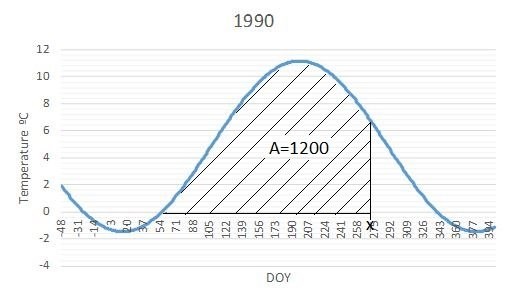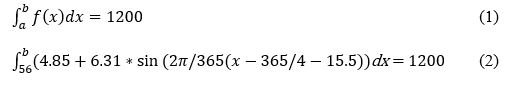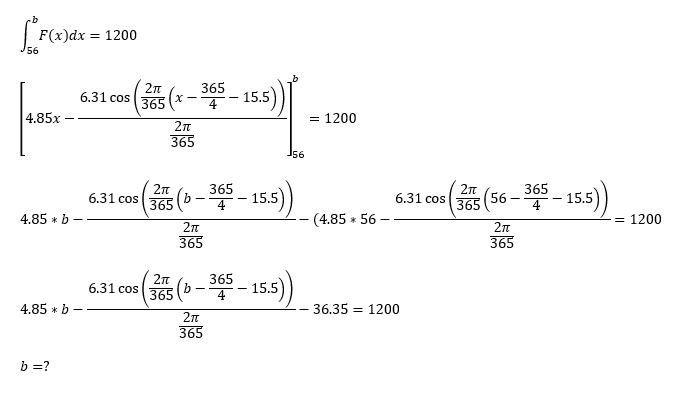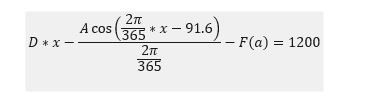## Recommended Posts

Hello,

I am modelling the length of the growth season for 120 different years based on estimated daily temperature. The temperature is estimated from a periodic function: f(x)=D+A*sin(B(x+C), where the constants B and C are the same every year and A and D varies.So I would like to know when the area under each function equals 1200 as shown in the figure. I think it can be solved by finding the upper limit b in equation 1 when the lower limit a is known. For the year 1990 it would look like equation 2, and b will take a value between a and 365. Do you know how I can calculate this using Excel?##### Share on other sites
Posted (edited)

Let $$F$$ be an antiderivative of your $$f;$$ $$F = \int f(x) dx.$$ That is easy, since $$f$$ is a simple trigonometric function. Then solve $$F(b)-F(a) = 1200$$ to determine $$a$$. Dunno what Excel can do. If it cannot do it, then use something else which can, like Maple or Mathematica, or code Newton-Raphson yourself. Once you have the solution, use Excel to document the correct solution to your boss.

Edited by taeto

##### Share on other sites
20 hours ago, taeto said:

Let F be an antiderivative of your f; F=f(x)dx. That is easy, since f is a simple trigonometric function. Then solve F(b)F(a)=1200 to determine a . Dunno what Excel can do. If it cannot do it, then use something else which can, like Maple or Mathematica, or code Newton-Raphson yourself. Once you have the solution, use Excel to document the correct solution to your boss.

Thanks a lot. Sadly, I have forgotten most of my highschool math and I haven't learned to code yet.  I tried already with CAS but the program keeps telling me that I need to specify and gives me another equation as the solution. Below are the steps I did for the year 1990. Is it wrong so far?Can I somehow isolate x in the equation below and specify that the answer is between a and 365?Kind regards,

##### Share on other sites
Posted (edited)

You are married too much to notation. You have to look at the form of the equation that you want to solve, which is $$x + \alpha \cos (\beta x + \gamma ) = c,$$ where you have known values for $$\alpha,\beta,\gamma, c.$$ You get that form by adding $$F(a)$$ on both sides of your last expression, and dividing through by $$D$$ on both sides. Or in other words, you want a solution to the equation $$h(x)=0,$$ where $$h(x) = x + \alpha \cos (\beta x + \gamma ) - c.$$ This cannot be solved as a closed expression, all you can do is to find an approximate solution. But so far as I can determine, your constants have values that are results of measurements, so they are themselves approximate? First try to make a drawing of the graph $$y = h(x)$$ to check if it is reasonable that there exists a solution to $$h(x) = 0$$ or even several solutions. If some value $$x_0$$ looks to be close to a solution, then use a software that is able to perform Newton's method for finding a zero of a function $$h(x)$$ close to a starting value $$x = x_0.$$ You may have to supply an expression for the derivative $$h'(x).$$ But since it is essentially a basic trigonometric function, this is an easy task.

Edited by taeto

## Create an account

Register a new account